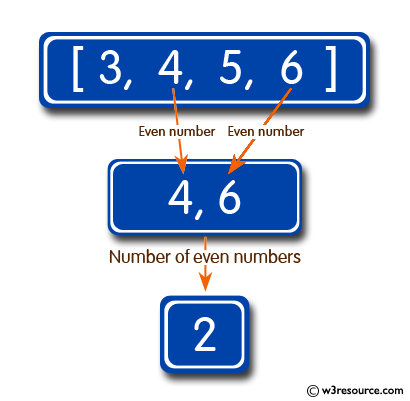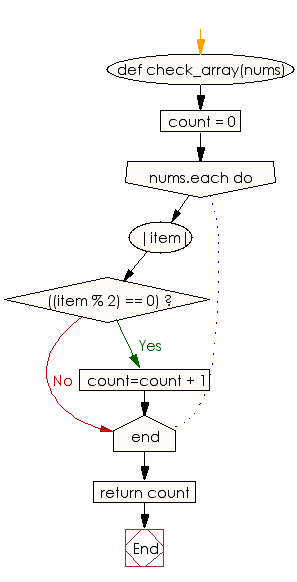﻿ Ruby Array exercises: Get the number of even integers in an given array - w3resource# Ruby Array Exercises: Get the number of even integers in a given array

## Ruby Array: Exercise-29 with Solution

Write a Ruby program to get the number of even integers in a given array.Ruby Code:

``````def check_array(nums)
count = 0
nums.each do |item|
if((item % 2) == 0)
count=count + 1
end
end
return count
end
print check_array([3, 4, 5, 6]),"\n"
print check_array([3, 4, 5]),"\n"
print check_array([3, 4])
``````

Output:

```2
1
1
```

Flowchart:Ruby Code Editor: